• 今天小编就为大家分享一篇Pytorch之卷积层的使用详解，具有很好的参考价值，希望对大家有所帮助。一起跟随小编过来看看吧
• 今天小编就为大家分享一篇Pytorch 实现冻结指定卷积层的参数，具有很好的参考价值，希望对大家有所帮助。一起跟随小编过来看看吧
• 今天小编就为大家分享一篇Pytorch卷积层手动初始化权值的实例，具有很好的参考价值，希望对大家有所帮助。一起跟随小编过来看看吧
• 今天小编就为大家分享一篇pytorch神经网络之卷积层与全连接层参数的设置方法，具有很好的参考价值，希望对大家有所帮助。一起跟随小编过来看看吧
• 将注意力机制整合到视觉中的最新趋势已导致研究人员重新考虑卷积层作为主要构建块的优势。 除了帮助CNN处理远程依赖关系之外，Ramachandran等人（英文）。 （2019）表明，注意力可以完全取代卷积，并在视觉任务上...Python
• ## 卷积层

千次阅读 2019-12-08 00:13:54
作用：对输入数据进行特征提取，对...上述图片介绍了卷积层两个优点及卷积层中间的计算过程。 实现：（基于Keras，详细可看链接） Conv2D层 keras.layers.convolutional.Conv2D(filters, kernel_size, strides=(1, 1...
作用：对输入数据进行特征提取，对输入数据中隐含的关联性进行抽象。 优点：一：局部连接；二：权值共享。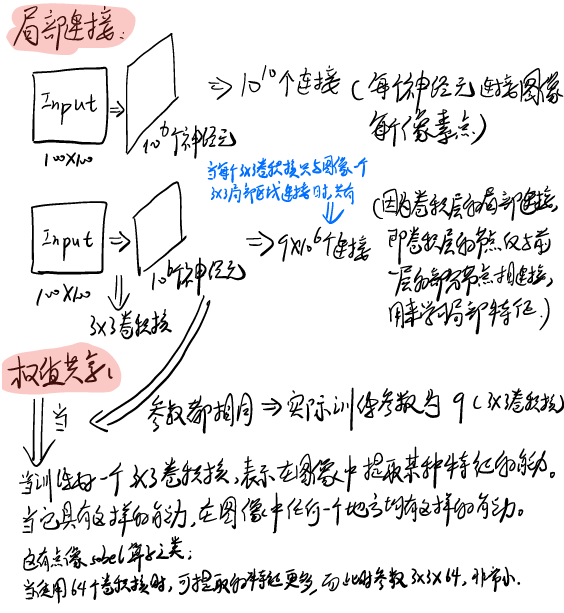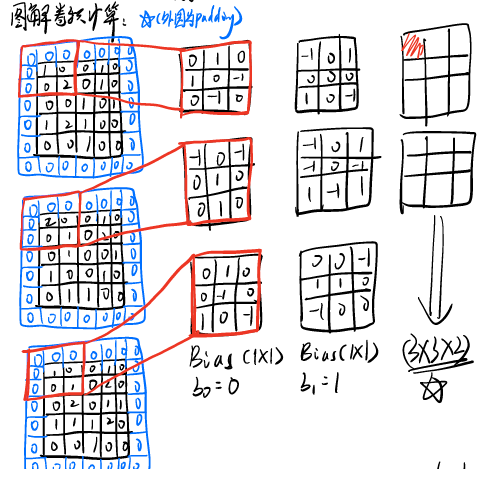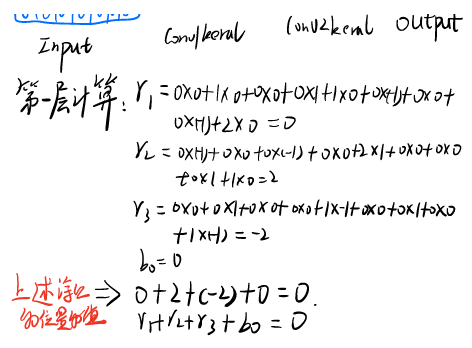（用Notability写的出现断页） 上述图片介绍了卷积层两个优点及卷积层中间的计算过程。 实现：（基于Keras，详细可看链接） Conv2D层 keras.layers.convolutional.Conv2D(filters, kernel_size, strides=(1, 1), padding=‘valid’, data_format=None, dilation_rate=(1, 1), activation=None, use_bias=True, kernel_initializer=‘glorot_uniform’, bias_initializer=‘zeros’, kernel_regularizer=None, bias_regularizer=None, activity_regularizer=None, kernel_constraint=None, bias_constraint=None)
二维卷积层，即对图像的空域卷积。该层对二维输入进行滑动窗卷积，当使用该层作为第一层时，应提供input_shape参数。例如input_shape = (128,128,3)代表128*128的彩色RGB图像（data_format=‘channels_last’） 参数 filters：卷积核的数目（即输出的维度） kernel_size：单个整数或由两个整数构成的list/tuple，卷积核的宽度和长度。如为单个整数，则表示在各个空间维度的相同长度。 strides：单个整数或由两个整数构成的list/tuple，为卷积的步长。如为单个整数，则表示在各个空间维度的相同步长。任何不为1的strides均与任何不为1的dilation_rate均不兼容 padding：补0策略，为“valid”, “same” 。“valid”代表只进行有效的卷积，即对边界数据不处理。“same”代表保留边界处的卷积结果，通常会导致输出shape与输入shape相同。 activation：激活函数，为预定义的激活函数名（参考激活函数），或逐元素（element-wise）的Theano函数。如果不指定该参数，将不会使用任何激活函数（即使用线性激活函数：a(x)=x） dilation_rate：单个整数或由两个个整数构成的list/tuple，指定dilated convolution中的膨胀比例。任何不为1的dilation_rate均与任何不为1的strides均不兼容。 data_format：字符串，“channels_first”或“channels_last”之一，代表图像的通道维的位置。该参数是Keras 1.x中的image_dim_ordering，“channels_last”对应原本的“tf”，“channels_first”对应原本的“th”。以128x128的RGB图像为例，“channels_first”应将数据组织为（3,128,128），而“channels_last”应将数据组织为（128,128,3）。该参数的默认值是~/.keras/keras.json中设置的值，若从未设置过，则为“channels_last”。 use_bias:布尔值，是否使用偏置项 kernel_initializer：权值初始化方法，为预定义初始化方法名的字符串，或用于初始化权重的初始化器。参考initializers bias_initializer：权值初始化方法，为预定义初始化方法名的字符串，或用于初始化权重的初始化器。参考initializers kernel_regularizer：施加在权重上的正则项，为Regularizer对象 bias_regularizer：施加在偏置向量上的正则项，为Regularizer对象 activity_regularizer：施加在输出上的正则项，为Regularizer对象 kernel_constraints：施加在权重上的约束项，为Constraints对象 bias_constraints：施加在偏置上的约束项，为Constraints对象 输入shape ‘channels_first’模式下，输入形如（samples,channels，rows，cols）的4D张量 ‘channels_last’模式下，输入形如（samples，rows，cols，channels）的4D张量 注意这里的输入shape指的是函数内部实现的输入shape，而非函数接口应指定的input_shape，请参考下面提供的例子。 输出shape ‘channels_first’模式下，为形如（samples，nb_filter, new_rows, new_cols）的4D张量 ‘channels_last’模式下，为形如（samples，new_rows, new_cols，nb_filter）的4D张量 输出的行列数可能会因为填充方法而改变 顺序模型中可以这样用model.add.conv2D (各个参数） 单独使用，conv2D(各个参数)
展开全文• 卷积层和完全连接的层进行过滤修剪的简单方法！ 您可以使用它来确定单个卷积和完全连接的层对修剪的敏感性。 共同研究者 也请访问我的共同研究人员的github！ training_initial_model.py 该脚本用于训练初始...Python
• 本文来自于云社区，本文章主要介绍了转置卷积层和正卷积层的关系和区别是什么呢，转置卷积层实现过程又是什么样的呢,希望读后会给您带来帮助。在CNN提出之前，我们所提到的人工神经网络应该多数情况下都是前馈神经...
• 修改了基层，在基层后加了一个预处理层，将数据归一化到500维的正确维度上
• ## 卷积层与池化层

万次阅读 多人点赞 2018-08-04 16:08:16
卷积神经网络（Convolutional Neural Layer, CNN)，除了全连接层以外（有时候也不含全连接层，因为出现了Global average pooling），还包含了卷积层和池化层。卷积层用来提取特征，而池化层可以减少参数数量。 ...
构建了最简单的网络之后，是时候再加上卷积和池化了。这篇，虽然我还没开始构思，但我知道，一定是很长的文章。
卷积神经网络（Convolutional Neural Layer, CNN)，除了全连接层以外（有时候也不含全连接层，因为出现了Global average pooling），还包含了卷积层和池化层。卷积层用来提取特征，而池化层可以减少参数数量。
卷积层
先谈一下卷积层的工作原理。
我们是使用卷积核来提取特征的，卷积核可以说是一个矩阵。假如我们设置一个卷积核为3*3的矩阵，而我们图片为一个分辨率5*5的图片。那么卷积核的任务就如下所示：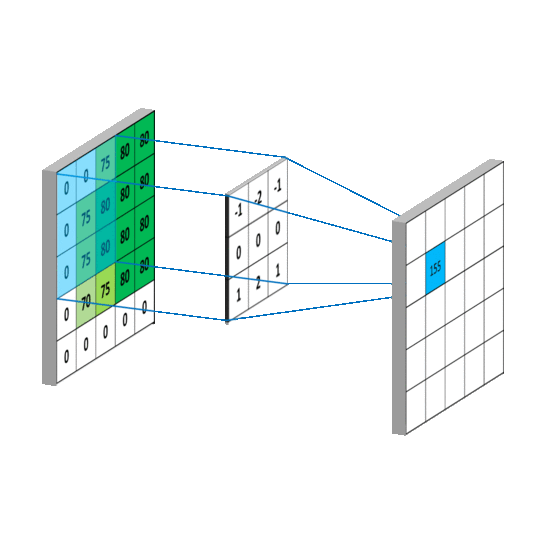来自：https://mlnotebook.github.io/post/CNN1/

从左上角开始，卷积核就对应着数据的3*3的矩阵范围，然后相乘再相加得出一个值。按照这种顺序，每隔一个像素就操作一次，我们就可以得出9个值。这九个值形成的矩阵被我们称作激活映射（Activation map)。这就是我们的卷积层工作原理。也可以参考下面一个gif:  其中，卷积核为

101010101

1

0

1

0

1

0

1

0

1

\begin{matrix}
1 & 0 & 1 \\
0 & 1 & 0 \\
1 & 0 & 1
\end{matrix}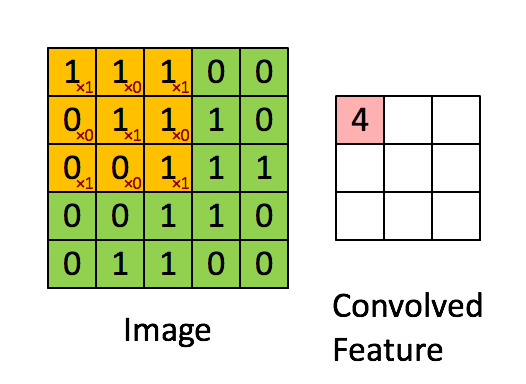来自：https://blog.csdn.net/yunpiao123456/article/details/52437794

其实我们平时举例的卷积核已经被翻转180度一次了，主要是因为计算过程的原因。详细不用了解，但原理都一样。

但其实我们输入的图像一般为三维，即含有R、G、B三个通道。但其实经过一个卷积核之后，三维会变成一维。它在一整个屏幕滑动的时候，其实会把三个通道的值都累加起来，最终只是输出一个一维矩阵。而多个卷积核(一个卷积层的卷积核数目是自己确定的）滑动之后形成的Activation Map堆叠起来，再经过一个激活函数就是一个卷积层的输出了。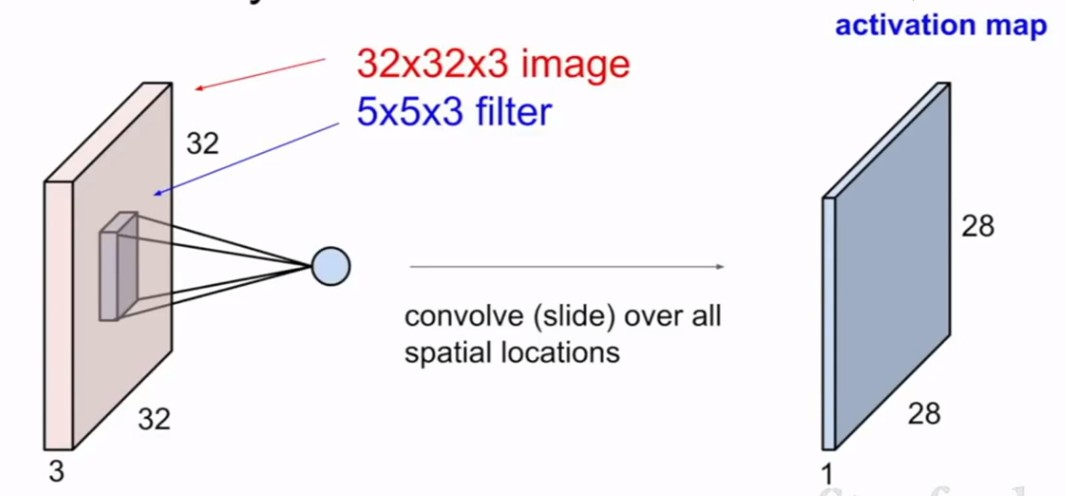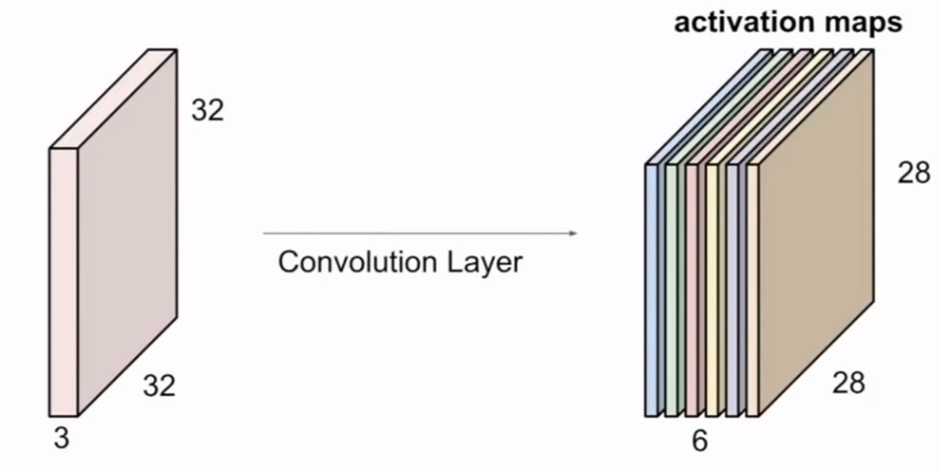来自：CS231n，卷积与池化

所谓的步长就是控制卷积核移动的距离。在上面的例子看到，卷积核都是隔着一个像素进行映射的，那么我们也可以让它隔着两个、三个，而这个距离被我们称作步长。
下面是示意图：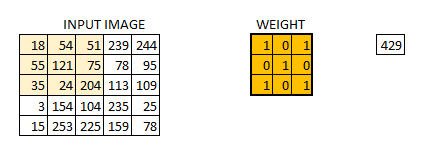步长为2 （图片来自于机器之心）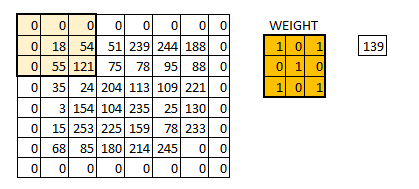补0的变化 （图片来自于机器之心）

了解卷积发展史的人都应该知道，卷积神经网络应用最开始出现是LeCun（名字真的很像中国人）在识别手写数字创建的LeNet-5。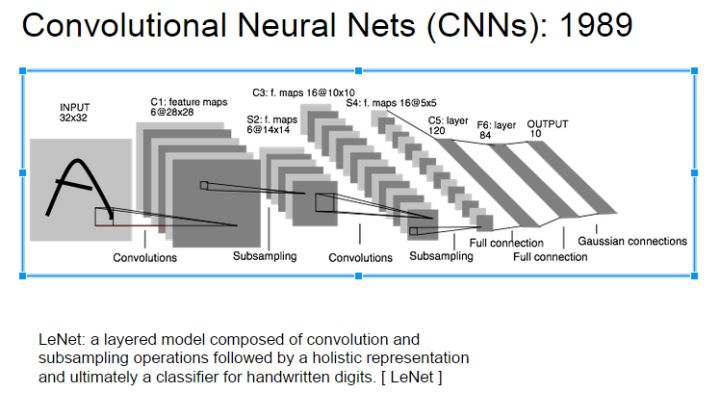后面爆发是因为AlexNet在ImageNet比赛中拔得头筹，硬生生把误差变成去年的一半。从此卷积网络就成了AI的大热点，一大堆论文和网络不断地发挥它的潜能，而它的黑盒性也不断被人解释。
能否对卷积神经网络工作原理做一个直观的解释？ - Owl of Minerva的回答 - 知乎里面通过我们对图像进行平滑的操作进而解释了卷积核如何读取特征的。
我们需要先明确一点，实验告诉我们人类视觉是先对图像边缘开始敏感的。在我的理解中，它就是说我们对现有事物的印象是我们先通过提取边界的特征，然后逐渐的完善再进行组装而成的。而我们的卷积层很好的做到了这一点。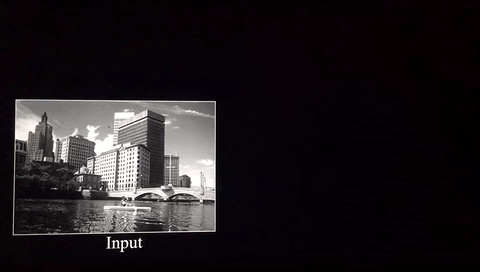来自https://ujjwalkarn.me/2016/08/11/intuitive-explanation-convnets/

这是两个不同的卷积核滑动整个图像后出来的效果，可以看出，经过卷积之后图像的边界变得更加直观。我们也可以来看下VGG-16网络第一层卷积提取到的特征: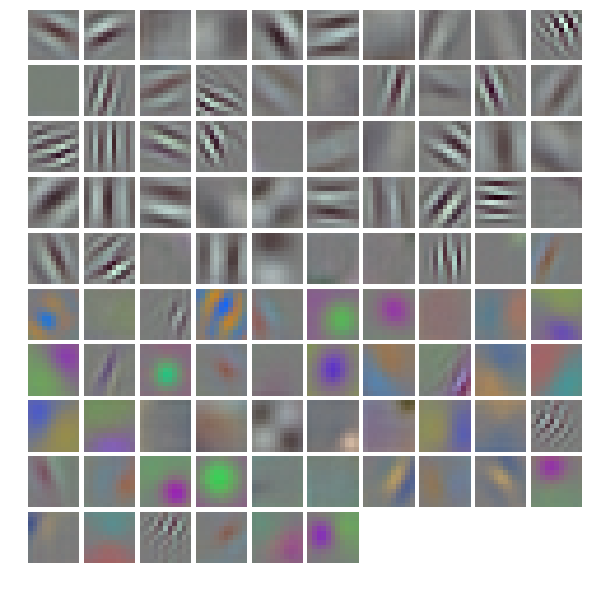由此来看，我们也知道为什么我们不能只要一个卷积核。在我的理解下，假使我们只有一个卷积核，那我们或许只能提取到一个边界。但假如我们有许多的卷积核检测不同的边界，不同的边界又构成不同的物体，这就是我们怎么从视觉图像检测物体的凭据了。所以，深度学习的“深”不仅仅是代表网络，也代表我们能检测的物体的深度。即越深，提取的特征也就越多。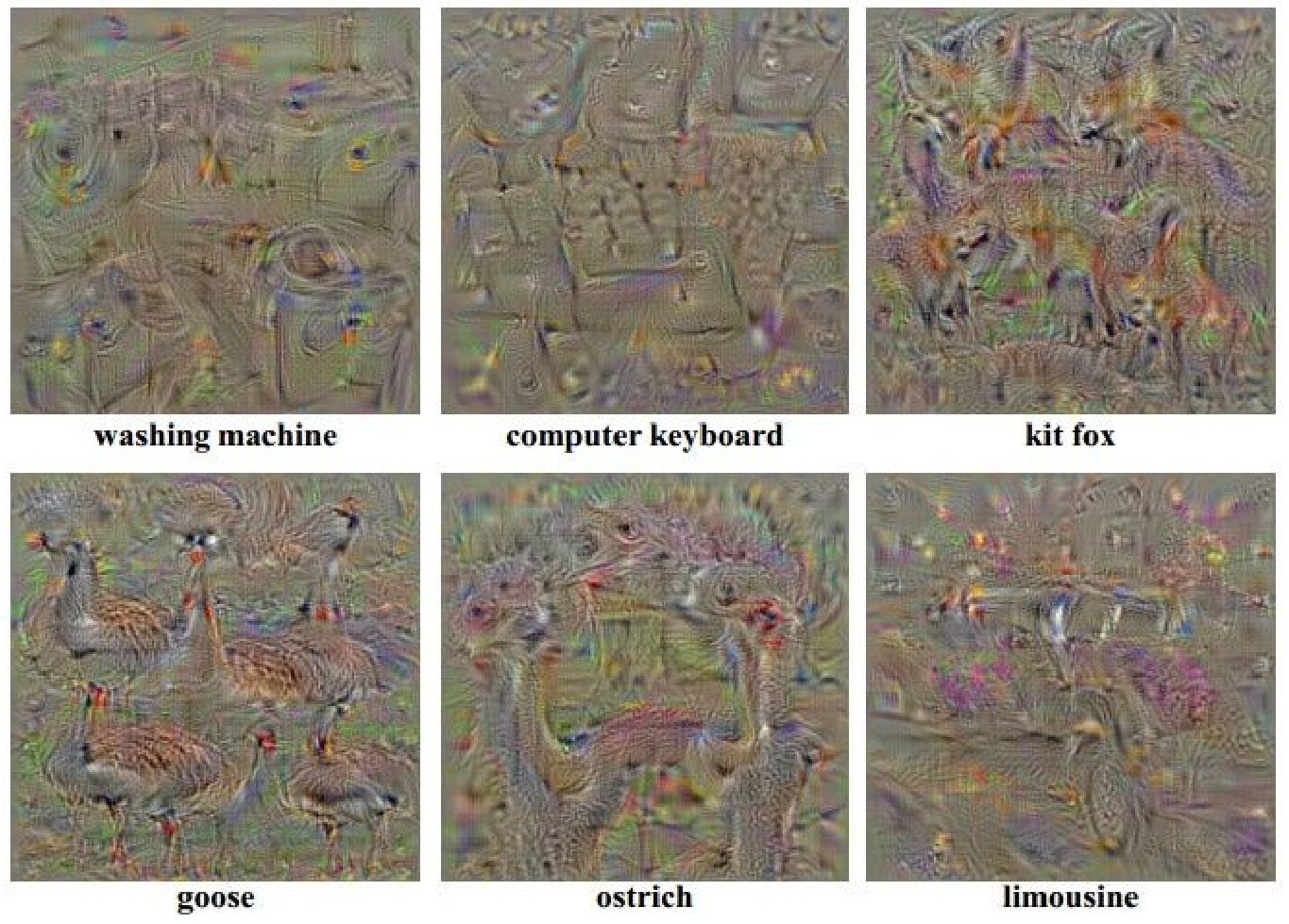其实这鹅看着还不错，有点像孔雀。

池化层 (pooling layer)
前面说到池化层是降低参数，而降低参数的方法当然也只有删除参数了。
一般我们有最大池化和平均池化，而最大池化就我认识来说是相对多的。需要注意的是，池化层一般放在卷积层后面。所以池化层池化的是卷积层的输出！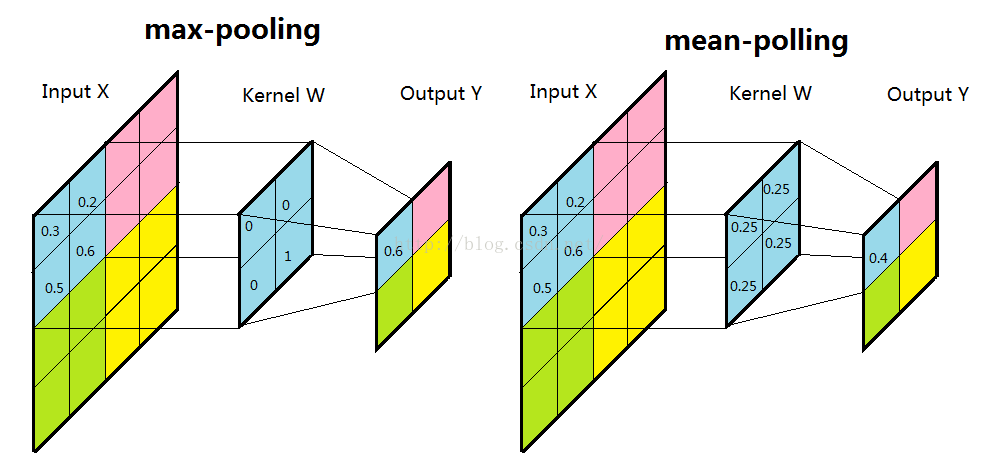来自：https://blog.csdn.net/yunpiao123456/article/details/52437794

扫描的顺序跟卷积一样，都是从左上角开始然后根据你设置的步长逐步扫描全局。有些人会很好奇最大池化的时候你怎么知道哪个是最大值，emmm，其实我也考虑过这个问题。CS2131n里面我记得是说会提前记录最大值保存在一个矩阵中，然后根据那个矩阵来提取最大值。
至于要深入到计算过程与否，应该是没有必要的。所以我也没去查证过程。而且给的都是示例图，其实具体的计算过程应该也是不同的，但效果我们可以知道就好了。
至于为什么选择最大池化，应该是为了提取最明显的特征，所以选用的最大池化。平均池化呢，就是顾及每一个像素，所以选择将所有的像素值都相加然后再平均。
代码解析
import tensorflow as tf
import numpy as np
from tensorflow.examples.tutorials.mnist import input_data

summary_dir = './summary'
#批次
batch_size = 100

n_batch = mnist.train.num_examples // batch_size

x = tf.placeholder(tf.float32, [None, 784], name='input')
y = tf.placeholder(tf.float32, [None, 10], name='label')

def net(input_tensor):
conv_weights = tf.get_variable('weight', [3, 3, 1, 32],
initializer=tf.truncated_normal_initializer(stddev=0.1))
conv_biases = tf.get_variable('biase', , initializer=tf.constant_initializer(0.0))

conv = tf.nn.conv2d(input_tensor, conv_weights, strides=[1, 1, 1, 1], padding='SAME')

pool = tf.nn.max_pool(relu, ksize=[1, 3, 3, 1], strides=[1, 2, 2, 1], padding='SAME')

pool_shape = pool.get_shape().as_list()
nodes = pool_shape * pool_shape * pool_shape
pool_reshaped = tf.reshape(pool, [-1, nodes])

W = tf.Variable(tf.zeros([nodes, 10]), name='weight')
b = tf.Variable(tf.zeros(), name='bias')
fc = tf.nn.softmax(tf.matmul(pool_reshaped, W) + b)

return fc

reshaped = tf.reshape(x, (-1, 28, 28, 1))
prediction = net(reshaped)
loss_ = tf.nn.sparse_softmax_cross_entropy_with_logits(labels=tf.argmax(y, 1), logits=prediction, name='loss')
loss = tf.reduce_mean(loss_)

correct_prediction = tf.equal(tf.argmax(y, 1), tf.argmax(prediction, 1))
accuracy = tf.reduce_mean(tf.cast(correct_prediction, tf.float32), name='accuracy')

init = tf.global_variables_initializer()

with tf.Session() as sess:
sess.run(init)
for epoch in range(31):
for batch in range(n_batch):
batch_xs, batch_ys = mnist.train.next_batch(batch_size)
sess.run(train_step, feed_dict={x: batch_xs, y: batch_ys})
acc = sess.run(accuracy, feed_dict={x: mnist.test.images, y: mnist.test.labels})
print('Iter' + str(epoch) + ",Testing Accuracy" + str(acc))
这相对于我第一个只用全连接的网络只多了一个net函数，还有因为卷积层的关系进来的数据x需要改变形状。只讲这两部分：
reshaped = tf.reshape(x, (-1, 28, 28, 1))
prediction = net(reshaped)
由于我们feedict上面是，feed_dict={x: mnist.test.images, y: mnist.test.labels}，而这样子调用tensorflow的句子我们得到的x是固定的形状。因此我们应用tf.reshape(x_need_reshaped,object_shape)来得到需要的形状。
其中的

−1

−

1

$-1$表示拉平，不能用None，是固定的。
conv_weights = tf.get_variable('weight', [3, 3, 1, 32],
initializer=tf.truncated_normal_initializer(stddev=0.1))
conv_biases = tf.get_variable('biase', , initializer=tf.constant_initializer(0.0))

conv = tf.nn.conv2d(input_tensor, conv_weights, strides=[1, 1, 1, 1], padding='SAME')

pool = tf.nn.max_pool(relu, ksize=[1, 3, 3, 1], strides=[1, 2, 2, 1], padding='SAME')
大部分都是应用内置的函数，来初始化weight(就是卷积核)和biases(偏置项)。偏置项我们没有提到，但其实就是多了一个参数来调控，因此我们讲卷积层的时候也没怎么讲。按照代码就是出来Activation Map之后再分别加上bias。池化也是用到了最大池化。
注意一下relu。它也是一个激活函数，作用可以说跟之前讲的softmax一样，不过它在卷积层用的比较多，而且也是公认的比较好的激活函数。它的变体有很多。有兴趣大家可以自己去查阅资料。以后才会写有关这方面的文章。
    pool_shape = pool.get_shape().as_list()
nodes = pool_shape * pool_shape * pool_shape
pool_reshaped = tf.reshape(pool, [-1, nodes])

W = tf.Variable(tf.zeros([nodes, 10]), name='weight')
池化层的输出我们并不知道它是如何的形状（当然，你也可以动手算）。因此就算把池化层拉成一维的矩阵，我们也不知道W需要如何的形状。因此，我们查看pool（即池化层的输出）的形状，我暗地里print了一下为[None, 14, 14, 32]，因此pool拉平后，就是[None, 14*14*32, 10]。为了接下来进行全连接层的计算，我们的W的形状也应该为[14*14*32, 10]。这段代码的原理就是如此。
准确率也一样取后15次：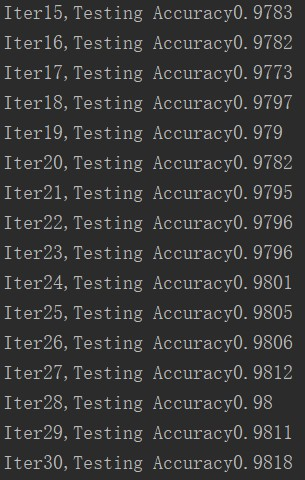emmm, 不用跟之前比了，明显比以前好很多了。下一章决定总结一下，优化的方法好了。

参考  https://mlnotebook.github.io/post/CNN1/（可惜是全英）  能否对卷积神经网络工作原理做一个直观的解释？ - Owl of Minerva的回答 - 知乎  CS231n

展开全文池化层 MNIST 提取特征 卷积原理
• 这些层是标准卷积层的直接替换（或添加）。 有关更多详细信息，请参见。 引文 原始论文可以通过以下方式引用： @misc{su2020arma, title={ARMA Nets: Expanding Receptive Field for Dense Prediction}, author...
• 主要介绍了keras中的卷积层&池化层的用法，具有很好的参考价值，希望对大家有所帮助。一起跟随小编过来看看吧
• 卷积层、反卷积层和相关层三个层之间有很大的相似之处，现在好好分析一下。 1.卷积层 下图较大网格表示一幅图片，有颜色填充的网格表示一个卷积核，卷积核的大小为33。假设我们做步长为1的卷积操作，表示卷积核...
卷积层、反卷积层和相关层三个层之间有很大的相似之处，现在好好分析一下。 1.卷积层 下图较大网格表示一幅图片，有颜色填充的网格表示一个卷积核，卷积核的大小为33。假设我们做步长为1的卷积操作，表示卷积核每次向右移动一个像素（当移动到边界时回到最左端并向下移动一个单位）。卷积核每个单元内有权重，下图的卷积核内有9个权重。在卷积核移动的过程中将图片上的像素和卷积核的对应权重相乘，最后将所有乘积相加得到一个输出。下图经过卷积后形成一个64的图。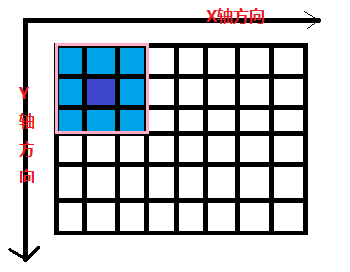在我们一般的网络中往往输入的图片是多个通道的（红绿蓝三个颜色通道），所以这是我们的卷积核也是三维的。比如我们的输入是28283的图片（前两个28代表图片的长和宽，后面的3代表三个颜色通道），这是我们的第一个卷积层所使用的卷积核是333的（卷积核的第三维通道数和图片的通道数一定是一样的，这里是三）;这是我们的步长为1,那么我们通过卷积操作就获取了一张26261的一张特征图。但是这时我们只提取了一次特征，在卷积神经网络中，为了更好的发挥网络的功能，我们会在一个卷积层中使用许多个同样尺寸的卷积核，比如这里我们使用128个卷积核，那么通过第一层我们就得到了128个26261的特征图，把他们叠在一起就得到了28283的特征图，这就是一个卷积层的输出。
2.相关层 卷积的操作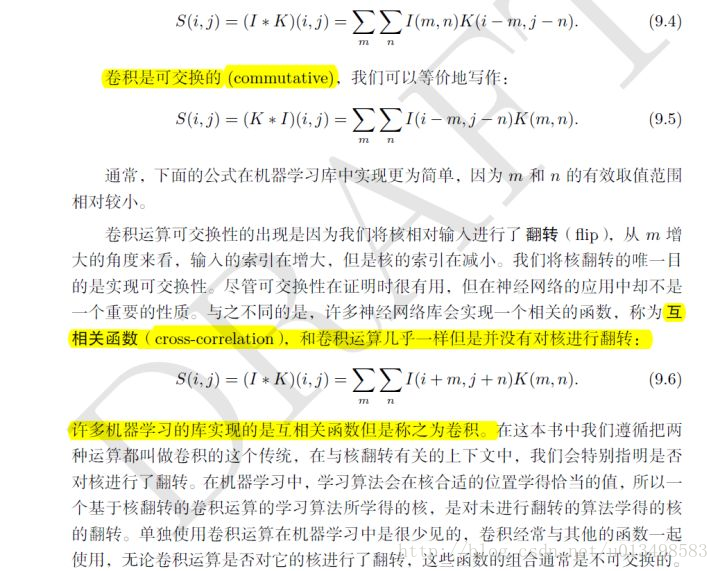也就是说，在卷积的过程中，其实我们是先将卷积核翻转180度之后，将它遍历整张图片进行点乘获取特征图。而在相关层中，其实我们的目的是找到两个特征图之间的相关程度。在计算时，它和卷积层的区别只在于它没有进行翻转。 对于两个特征图之间的相关层计算我们以下图的相关层为例。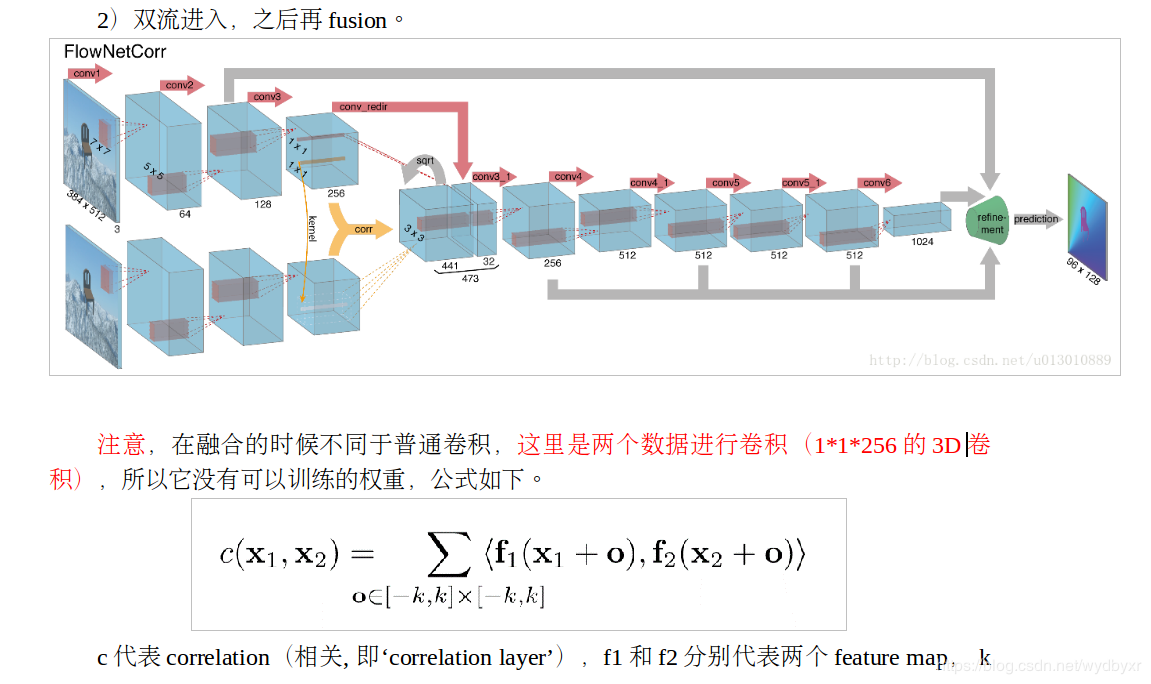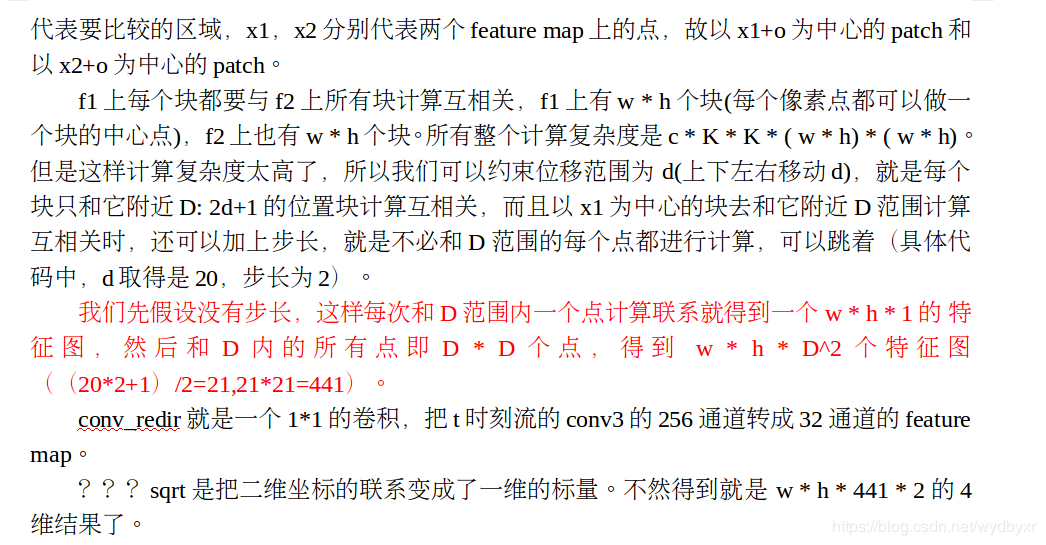在这个网络中，我们的输入是两张图片，我们将两张图片分别通过两个卷积神经网络，加入最后得到了两个384512256（256是通道数）的特征图，下一步我们的目的是找出两个特征图的相关度。具体的计算过程是首先我们上面的特征图中我们要将其中的384512256的特征找出384512个11256的卷积核进行相关层操作，根据上文，为了简化计算量，我们每个卷积核只和下面的特征图的一部分区域进行相关层操作。比如我们选择的尺度为10,那么每个卷积核将进行的相关层操作范围为2121（在384512的平面中以该点为中心，上下左右各取10）得到的操作范围作为获取的新的特征图的第三维数据，所以第三维数据为2121=441，一共有384512个卷积核，所以获取的特征图尺寸为384512441，再使用32个11256的卷积核获取了38451232的特征图，叠在一起就是384512473的特征图。 3.反卷积层 反卷积层并不是卷积层的逆运算，我认为它属于卷积层的一种变体。但它的任务与卷积层的不同在于卷积层的操作过程中会将图片的尺寸变小或保持不变，而反卷积层的任务是将特征图尺寸变大以实现全卷积神经网络的目的（往往我们会使用它来实现输入为图片，输出也是图片的任务）。卷积层的过程就是使用卷积核翻转后在原图片上进行遍历点乘，而反卷积层由于需要放大特征图的尺寸，所以我们首先会使用padding的方法，对特征图进行填充（一般补充零），进一步进行卷积操作。比如一个443的特征图,我们首先会对他进行填充，通过补0的方式将它变为883的特征图，进一步使用28个333的卷积核对它进行卷积操作，我们就得到了66*28的特征图，很好的解决了扩充图片尺寸的任务，同时提取了图片的特征。 填充的方法有很多种，如下图：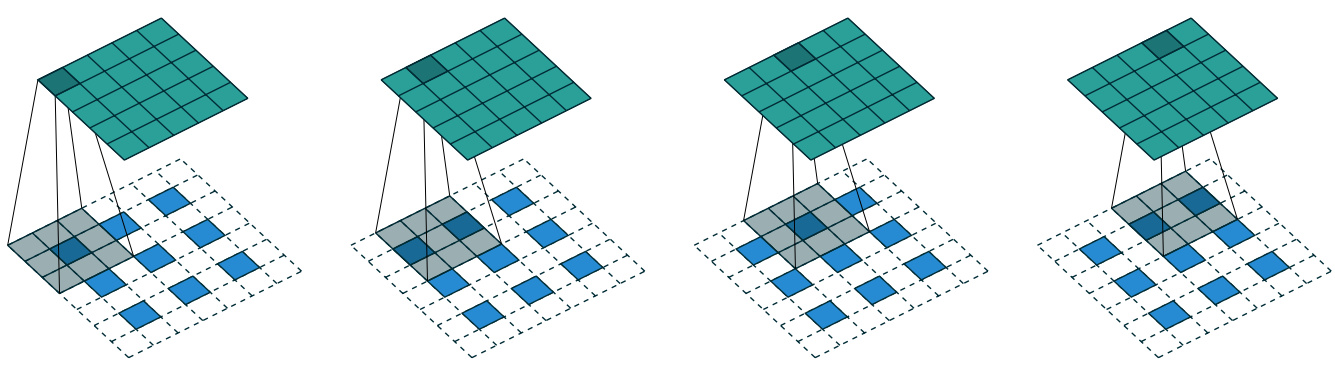但是填充时我们也并不是进行填充0，往往需要进行插值等方式来填充，如下图：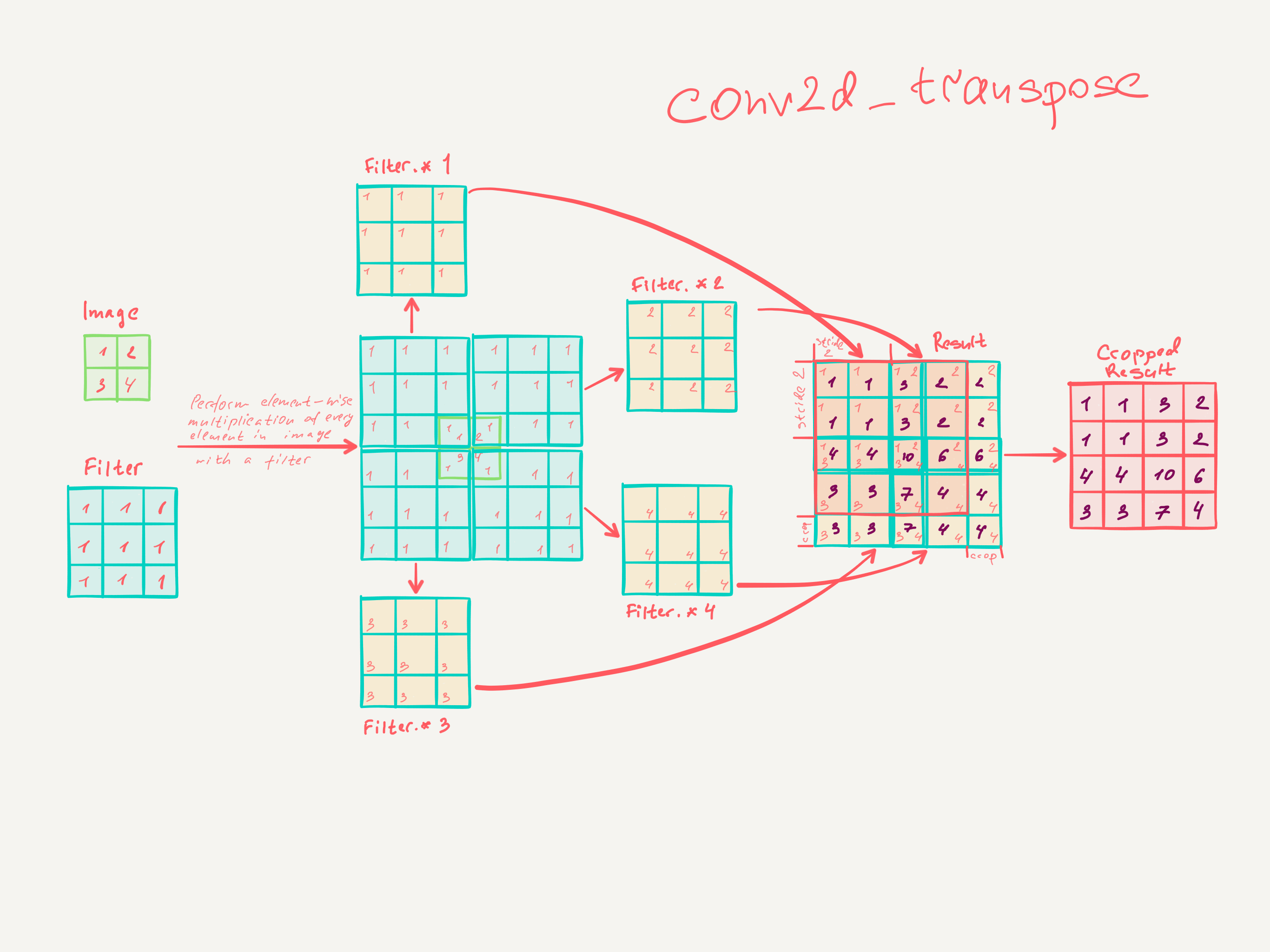参考：https://blog.csdn.net/Nianzu_Ethan_Zheng/article/details/79017711
展开全文卷积神经网络
• ## 卷积层TSNE可视化

千次阅读 热门讨论 2021-07-08 10:34:37
很多小伙伴经常问，怎么把卷积层的输出提取出来，然后画曲线、可视化、连接到其他网络等等问题，由于本人使用的是基于keras和tensorflow框架的Spyder软件编写的代码，因此对别的软件怎么输出参数不清楚，单说spyder...
很多小伙伴经常问，怎么把卷积层的输出提取出来，然后画曲线、可视化、连接到其他网络等等问题，由于本人使用的是基于keras和tensorflow框架的Spyder软件编写的代码，因此对别的软件怎么输出参数不清楚，单说spyder，往往经过卷积层后提取到的特征形式是：样本数量×特征长度×特征维度，因此即使输出来也是很操蛋的就一个样本长度为特征长度而且仅仅第一个维度的数据。使用这些数据去绘图很难，因为输出来的一个样本的数据并不是一条，而是经过堆叠成的数据。 但用这些数据来做一些可视化和连接到其他网络还是可以的。 以可视化为例，前面有过卷积层可视化，但并不能从图中得到有效信息。因此用这些数据做TSNE可视化试试看。由于卷积层输出来的数据是样本数量×特征长度×特征维度，而tsne输入是样本数乘特征数，因此需要将：样本数量×特征长度×特征维度重新reshape成样本数×特征数的形式。先看一下效果图，首先展示神经网络对数据进行二分类，观察每个卷积层的TSNE可视化情况： 1、原始数据TSNE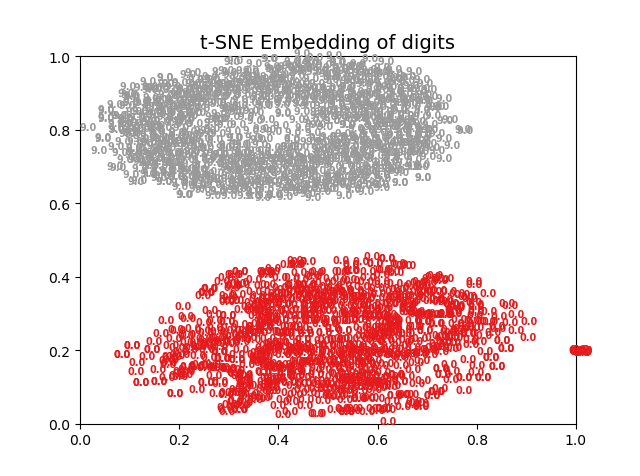2、第一个卷积层等不在意义叙述：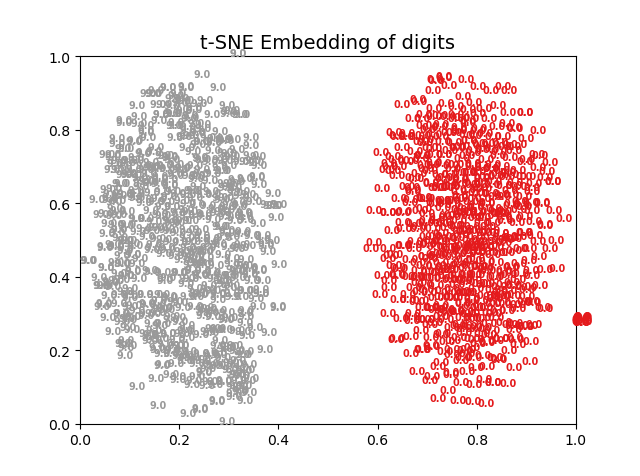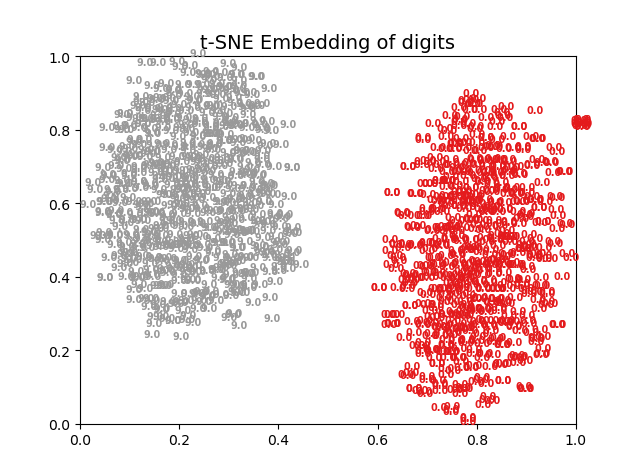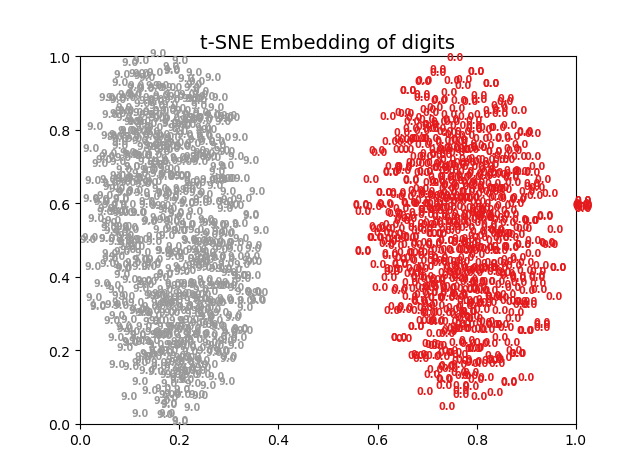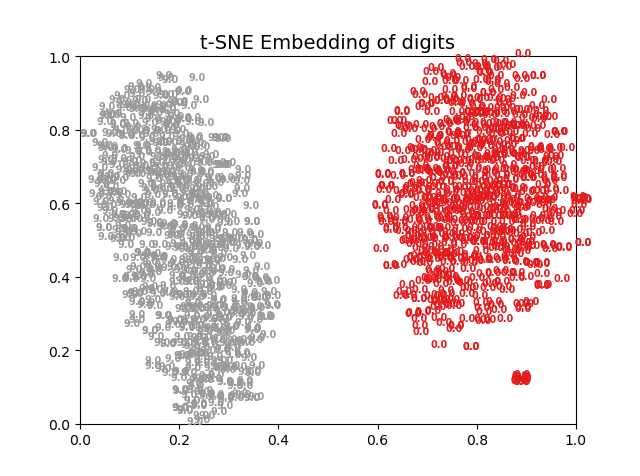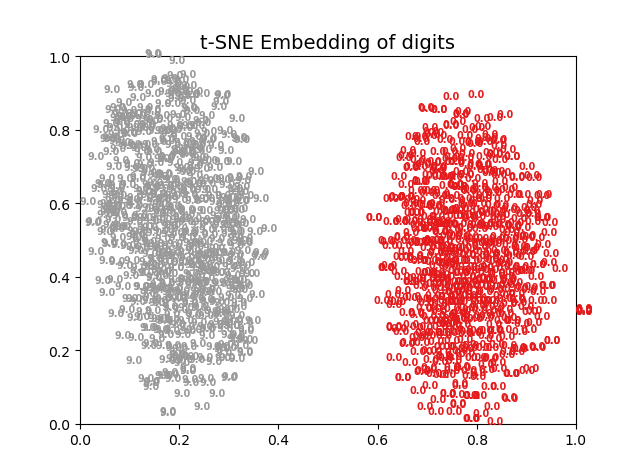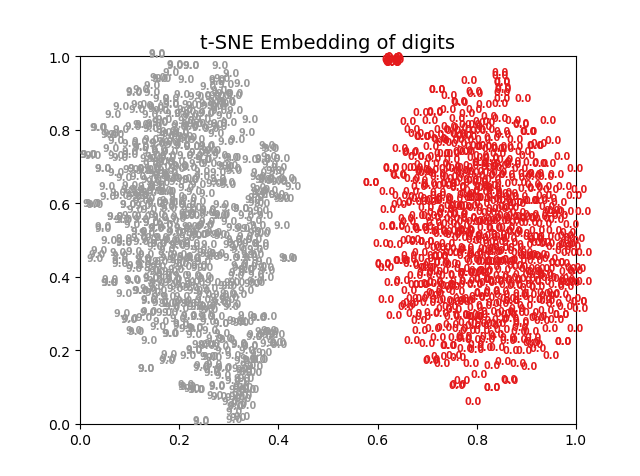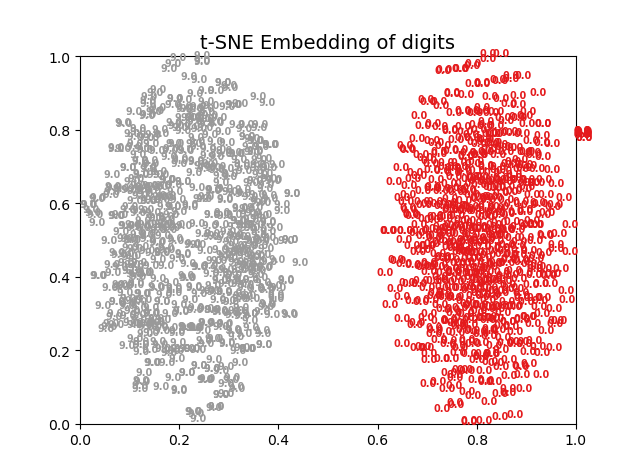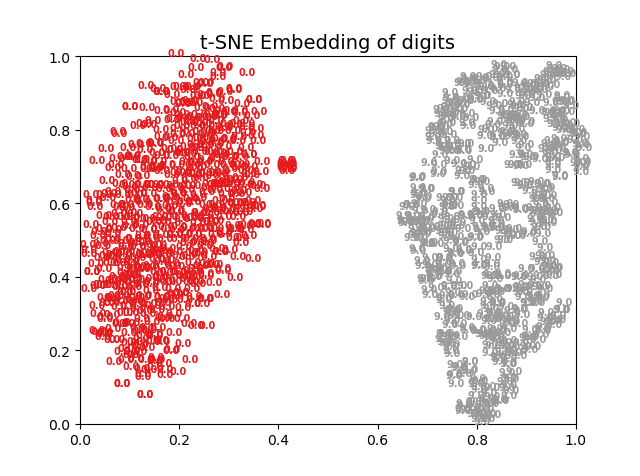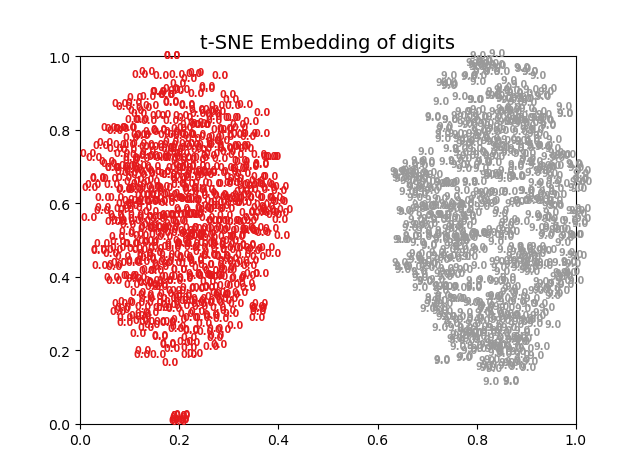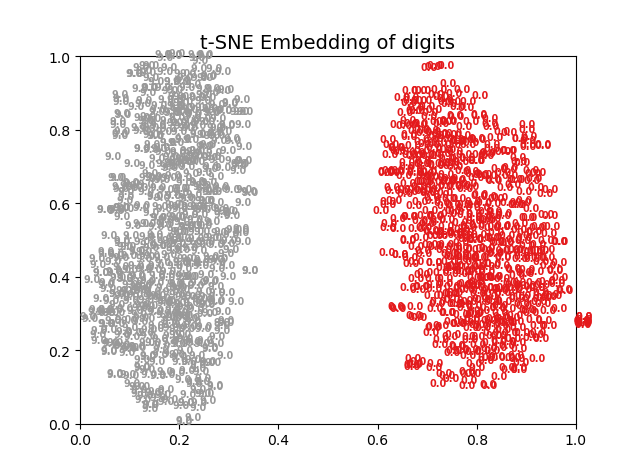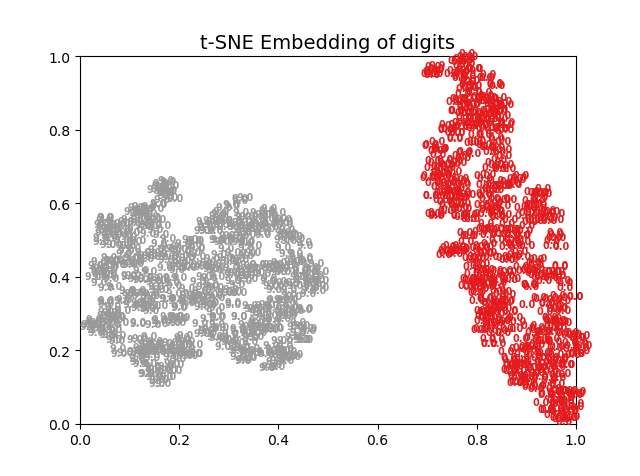从这些图能分析出什么？毛都分析不出来，仅仅能说明两种数据本来差异性就很大。另外就是，用神经网络做二分类完全没有展现出神经网络特征提取的强大能力，因此前面写的里面曲线收敛的非常快，差不多第二批次就收敛了，这也就没什么奇怪的了。
因此增加样本数量，并研究单一个卷积层在不同训练批次下，输出数据的可视化i情况： 1、没有训练：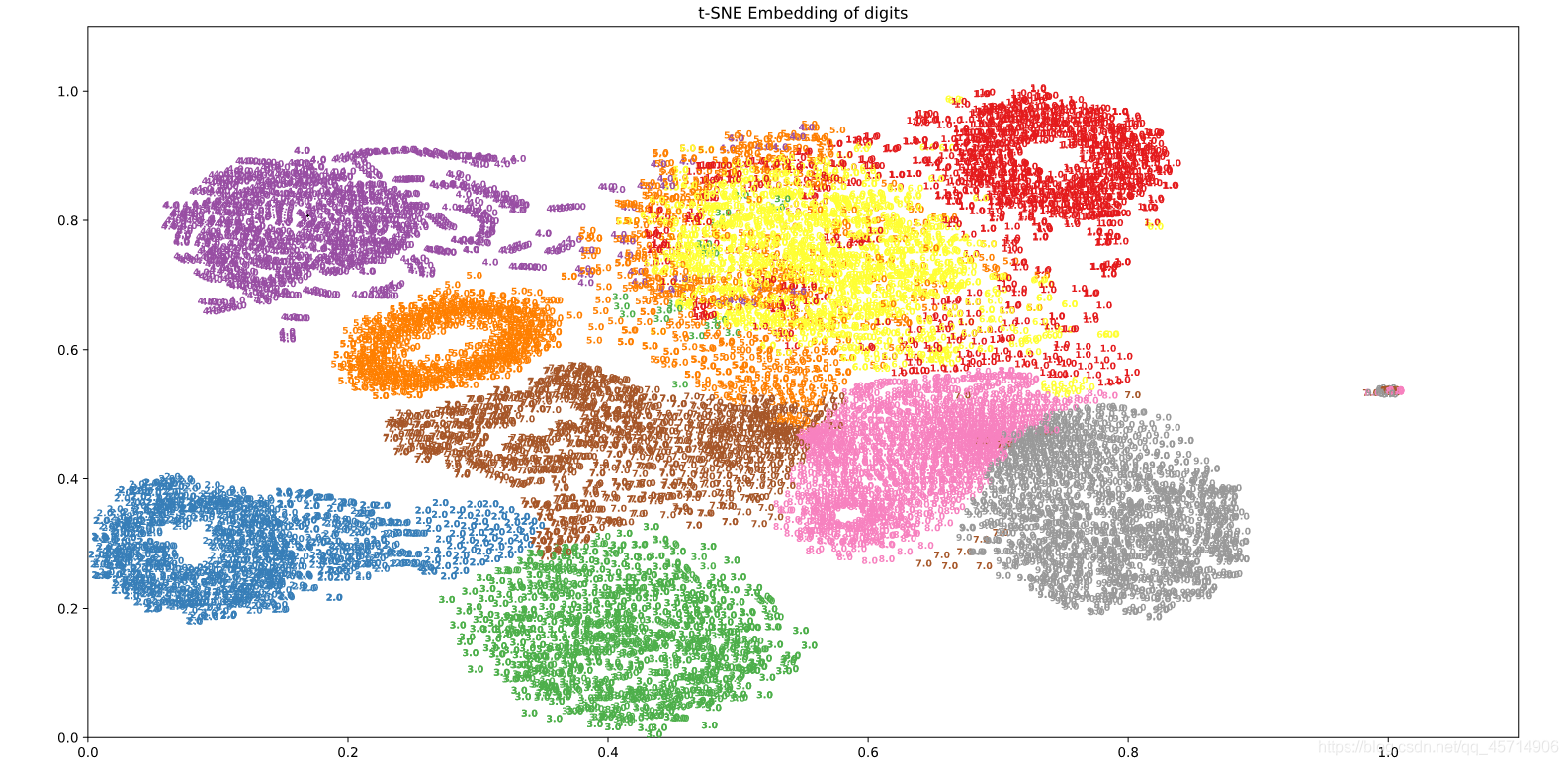2、训练3次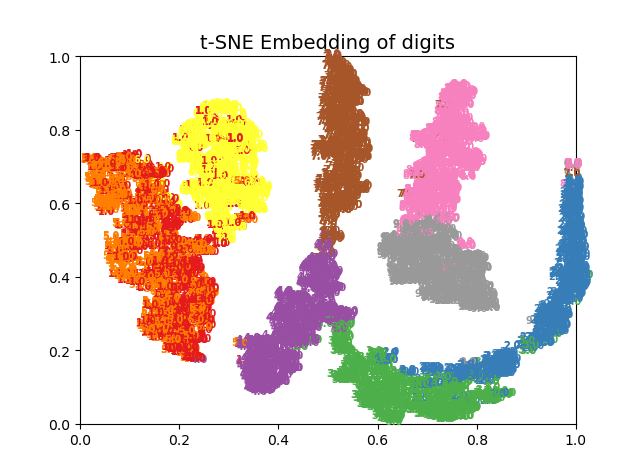3、训练300次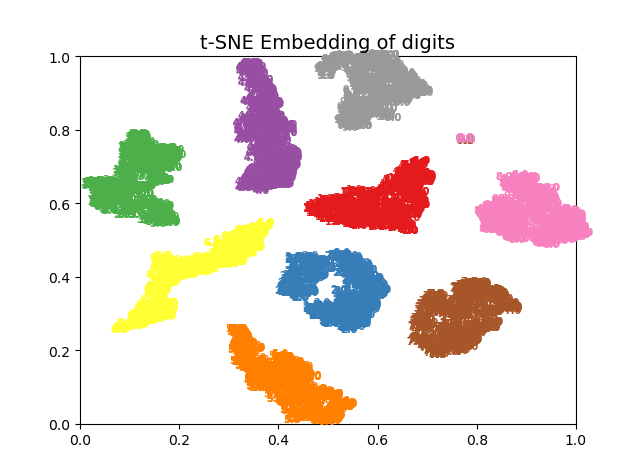4、训练3000次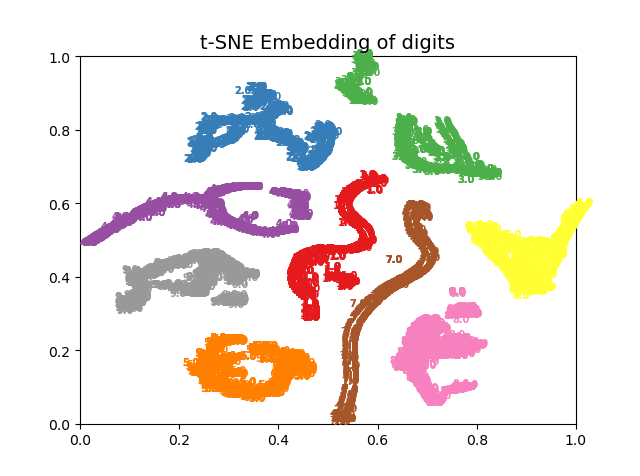这个效果就不用再赘述了，很明显训练300次最佳。
代码如下：
# -*- coding: utf-8 -*-
"""
Created on Wed Jul  7 11:55:08 2021

@author: 1
"""
import tensorflow as tf
from sklearn.manifold import TSNE
import numpy as np
import pandas as pd
import keras
from keras.models import Sequential
from keras.wrappers.scikit_learn import KerasClassifier
from keras.utils import np_utils,plot_model
from sklearn.model_selection import cross_val_score,train_test_split,KFold
from sklearn.preprocessing import LabelEncoder
from keras.models import model_from_json
import matplotlib.pyplot as plt
from sklearn.metrics import confusion_matrix
import itertools
from keras.optimizers import SGD
from keras.layers import Dense,LSTM, Activation, Flatten, Convolution1D, Dropout,MaxPooling1D,BatchNormalization
from sklearn import preprocessing
# 载入数据
X = np.expand_dims(df.values[:, 0:1024].astype(float), axis=2)
Y = df.values[:, 1024]

# 湿度分类编码为数字

# 划分训练集，测试集
X_train, X_test, K, y = train_test_split(X, Y, test_size=0.3, random_state=0)
K=K

encoder = LabelEncoder()
Y_encoded1 = encoder.fit_transform(K)
Y_train = np_utils.to_categorical(Y_encoded1)

Y_encoded2 = encoder.fit_transform(y)
Y_test = np_utils.to_categorical(Y_encoded2)

# 定义神经网络
def baseline_model():
model = Sequential()
model.add(BatchNormalization(axis=-1, momentum=0.99, epsilon=0.001, center=True, scale=True, beta_initializer='zeros', gamma_initializer='ones', moving_mean_initializer='zeros', moving_variance_initializer='ones', beta_regularizer=None, gamma_regularizer=None, beta_constraint=None, gamma_constraint=None))

model.add(BatchNormalization(axis=-1, momentum=0.99, epsilon=0.001, center=True, scale=True, beta_initializer='zeros', gamma_initializer='ones', moving_mean_initializer='zeros', moving_variance_initializer='ones', beta_regularizer=None, gamma_regularizer=None, beta_constraint=None, gamma_constraint=None))

model.add(BatchNormalization(axis=-1, momentum=0.99, epsilon=0.001, center=True, scale=True, beta_initializer='zeros', gamma_initializer='ones', moving_mean_initializer='zeros', moving_variance_initializer='ones', beta_regularizer=None, gamma_regularizer=None, beta_constraint=None, gamma_constraint=None))

model.add(BatchNormalization(axis=-1, momentum=0.99, epsilon=0.001, center=True, scale=True, beta_initializer='zeros', gamma_initializer='ones', moving_mean_initializer='zeros', moving_variance_initializer='ones', beta_regularizer=None, gamma_regularizer=None, beta_constraint=None, gamma_constraint=None))

model.add(BatchNormalization(axis=-1, momentum=0.99, epsilon=0.001, center=True, scale=True, beta_initializer='zeros', gamma_initializer='ones', moving_mean_initializer='zeros', moving_variance_initializer='ones', beta_regularizer=None, gamma_regularizer=None, beta_constraint=None, gamma_constraint=None))

model.add(BatchNormalization(axis=-1, momentum=0.99, epsilon=0.001, center=True, scale=True, beta_initializer='zeros', gamma_initializer='ones', moving_mean_initializer='zeros', moving_variance_initializer='ones', beta_regularizer=None, gamma_regularizer=None, beta_constraint=None, gamma_constraint=None))

model.summary()
return model

# 训练分类器
estimator = KerasClassifier(build_fn=baseline_model, epochs=3000, batch_size=128, verbose=1)
history=estimator.fit(X_train, Y_train, validation_data=(X_test, Y_test))
import matplotlib.pyplot as plt

# 卷积网络可视化
def visual(model, data, num_layer=1):
layer = keras.backend.function([model.layers.input], [model.layers[num_layer].output])
f1 = layer([data])
np.set_printoptions(threshold=np.inf)
print(f1.shape)
print(f1)
f2=f1.reshape(6034,64)
print(f2)
num = f1.shape[-1]
print(num)
plt.figure(figsize=(6, 12), dpi=150)
for i in range(num):
plt.subplot(np.ceil(np.sqrt(num)), np.ceil(np.sqrt(num)), i+1)
plt.imshow(f1[:, :, i] * 255, cmap='prism')
plt.axis('off')
plt.show()
def get_data():

digits=2
data = f2#digits.data		# 图片特征
label = K#digits.target		# 图片标签
n_samples=6034
n_features =64 #data.shape		# 数据集的形状
return data, label, n_samples, n_features

# 对样本进行预处理并画图
def plot_embedding(data, label, title):

x_min, x_max = np.min(data, 0), np.max(data, 0)
data = (data - x_min) / (x_max - x_min)		# 对数据进行归一化处理
fig = plt.figure()		# 创建图形实例
ax = plt.subplot(111)		# 创建子图
# 遍历所有样本
for i in range(data.shape):
# 在图中为每个数据点画出标签
plt.text(data[i, 0], data[i, 1], str(label[i]), color=plt.cm.Set1(label[i] / 10),
fontdict={'weight': 'bold', 'size': 7})
plt.xticks()		# 指定坐标的刻度
plt.yticks()
plt.title(title, fontsize=14)
# 返回值
return fig

data, label , n_samples, n_features = get_data()		# 调用函数，获取数据集信息
print('Starting compute t-SNE Embedding...')
ts = TSNE(n_components=2, init='pca', random_state=0)
# t-SNE降维
reslut = ts.fit_transform(data)
# 调用函数，绘制图像
fig = plot_embedding(reslut, label, 't-SNE Embedding of digits')
# 显示图像
plt.show()

# 可视化卷积层
visual(estimator.model, X_train, 20)#在这里插入代码片


本来我是想拿这个代码作为创新点写个核心的，结果把把被拒说没有创新，么的办法只能放弃这个追求更高的创新了。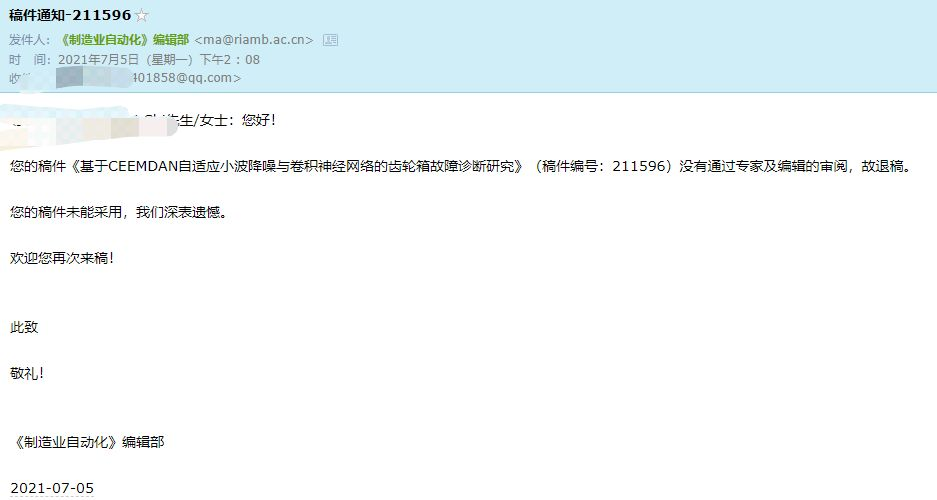我发现投稿的难度并不和这个期刊的影响因子成正比，今年好几个影响因子接近1的期刊被踢出北大核心了，而且例如机械设计与研究等期刊，影响因子高于1非常好中，但是像机械强度、制造业自动化等等影响因子小于0.5的期刊，纯看命，很难中。
本次所使用的数据如下连接，永久有效： 链接：https://pan.baidu.com/s/1jmOOKXFA27I6iGlvvMBiwg 提取码：SLBY 链接：https://pan.baidu.com/s/1plW9siresvTIvlNZsDA_tw 提取码：SLBY 两个数据名字一样，但是内容不一样。
展开全文可视化 深度学习 tensorflow pytorch
• ## 漫谈卷积层

千次阅读 2019-03-28 20:15:39
漫谈卷积层 原文链接：https://hey-yahei.cn/2019/03/28/Conv-Family/ 去年刚入手深度学习的时候写过一篇《卷积神经网络CNN | Hey~YaHei!》，简单介绍过卷积层——不过经过一年的学习和实践，对卷积又有了新的认识...深度学习 人工智能 卷积
• ## 卷积层详述

千次阅读 2019-10-16 16:42:24
这个就是卷积神经网络中卷积层最基础的运算。在实际的操作中，还存在一些额外的操作，下面会详细叙述。 该图出自： Convolutional Neural Networks - Basics 输入层 在使用卷积神经网络进行图像识别时，输入...
• 而对于常见的卷积神经网络，我们经常可以看到卷积层、池化层、激活函数、全连接层的身影。直到现在，卷积层+池化层+激活函数+全连接层的结构仍然很实用，似乎已经成为搭建卷积神经网络的基本结构。下面我将从这四个...神经网络搭建 池化 激活函数 全连接层
• CNN中的卷积层 直接实现方法 Caffe实现方法 FFT实现方法 CUDNN实现方法 FPGA硬件实现 优化策略
• 卷积层（convolutional layer） 1.卷积核（convolutional kernel） 卷积层的功能是对输入数据进行特征提取，其内部包含多个卷积核，组成卷积核的每个元素都对应一个权重系数和一个偏差量（bias vector），类似于一个...
• 基于matlab的神经网络中的卷积层基于matlab的神经网络中的卷积层MATLAB
• 卷积层 1、1d/2d/3d （1）卷积运算：卷积核在输入信号（图像）上滑动，相应位置上进行乘加 （2）卷积核：又称为滤波器，过滤器，可认为是某种模式，某种特征。 （3）卷积过程类似于用一个模版去图像上寻找与它相似的...pytorch 卷积 深度学习 计算机视觉 可视化...